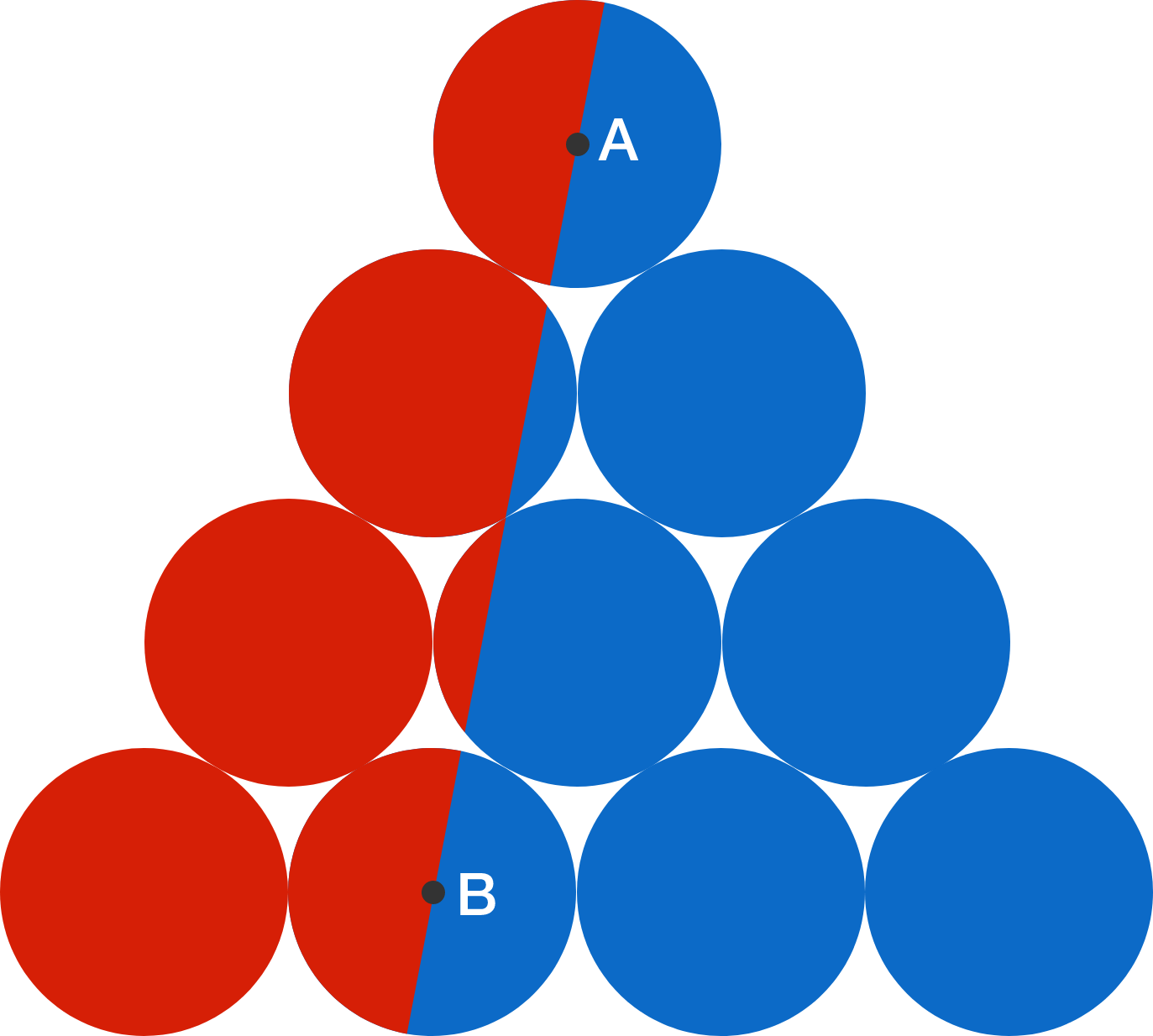# Divide 10 circles

Geometry Level 1

10 identical circles are stacked in a pyramid shape, where $A$ and $B$ are centers of two of the circles. A line is drawn through $A$ and $B,$ dividing the figure into 2 areas: red on the left and and blue on the right.

What is the ratio of the red area to the blue area?×

Problem Loading...

Note Loading...

Set Loading...